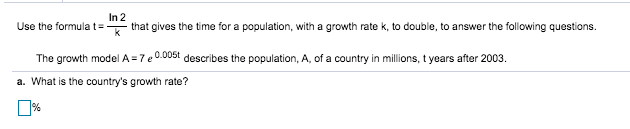# In 2that gives the time for a population, with a growth rate k, to double, to answer the following questions.Use the formulatkdescribes the population, A, of a country in millions, t years after 2003The growth model A 7ea. What is the country's growth rate?%

Question
1 viewshelp_outlineImage TranscriptioncloseIn 2 that gives the time for a population, with a growth rate k, to double, to answer the following questions. Use the formulat k describes the population, A, of a country in millions, t years after 2003 The growth model A 7e a. What is the country's growth rate? % fullscreen
check_circle

Step 1

The growth model of the population  and ...

### Want to see the full answer?

See Solution

#### Want to see this answer and more?

Solutions are written by subject experts who are available 24/7. Questions are typically answered within 1 hour.*

See Solution
*Response times may vary by subject and question.
Tagged in

### Applications of Mathematics